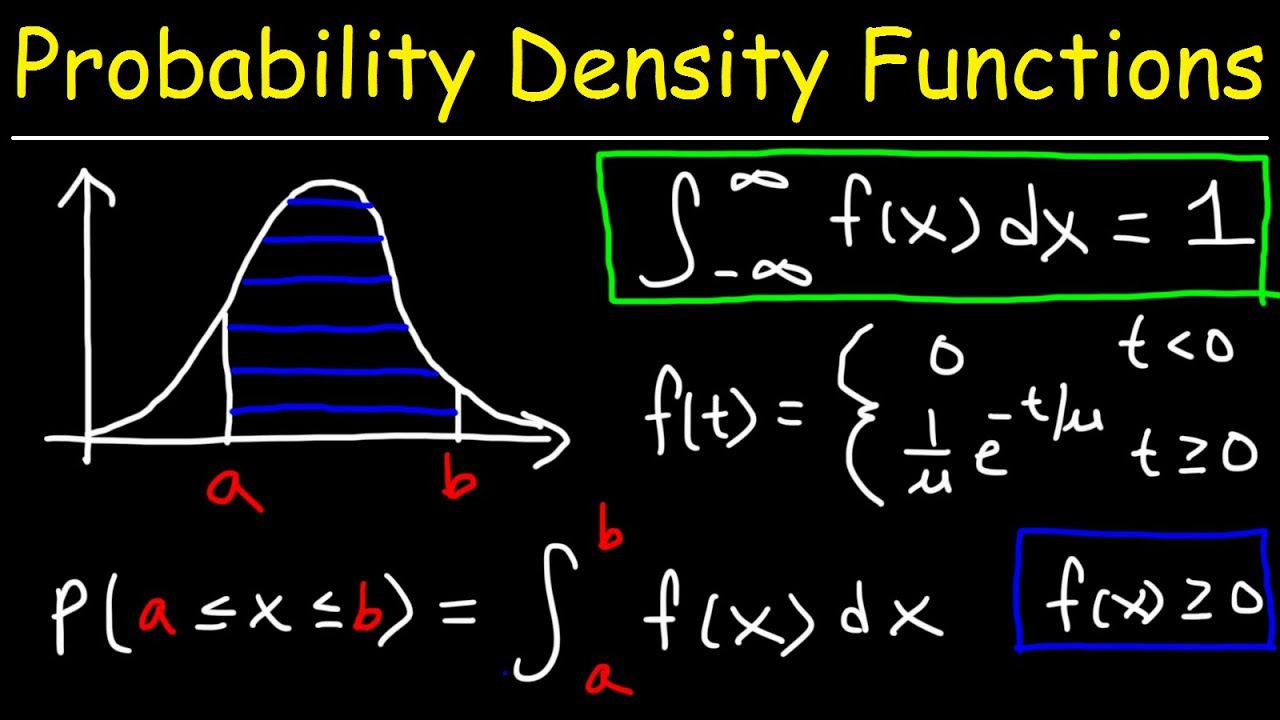# Week 9 11

Application-level and advanced high-level topics in Machine Learning

## Anomaly Detection System

### Gaussian Distribution

Anomaly detection uses gaussian distribution - probability density function formulaThe hypothesis model uses density estimation, the product of all density functions $p(x)$, to detect fraudsThe concept here is deeply incorporated into another concept likelihood - the anonymous data points usually have low likelihood

Likewise, anonymous data points usually have low value in density estimation function

### Feature engineering

Instead of $x_{new} = \frac{x-\mu}{\sigma}$, one can do log transform or change the degree of feature to form gaussian-like shape to make model happy e.g.

$x_{new} = log(x + 1)$, or $x_{new} = x^{0.2}$

Sometimes $p(x)$ is not that comparable (say, both large) for normal and anonymous data.

• To solve this problem, we can define new features e.g. $x_3 = {x_1}^2/x_2$ which can help capture unusually large or small values (outliers)

### Multivariate Gaussian Distribution

Motivation: what if normal data points cluster don't follow standard gaussian distribution shape - normal data are not within perfect circle but oval instead even when features are normalized (when feature engineering can't help a lot).

e.g. normal (red) vs. anonymous (green) - we can't draw circle (pink) bound to separate two classifications but need to draw oval (blue) bound belowModel hypothesis:Similar to normal gaussian distribution step, to detect anonymous data using multivariate gaussian distribution, we plug data into model and use a threshold $\epsilon$### Multivariate vs. single variate

So multivariate gaussian model basically is a more general form with flexible covariance matrix $\sum$, where original normal gaussian model requires $\sum$ to be diagonal## Large Scale Machine Learning

Different names for different kind of gradient descents